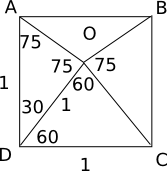Question 25

# An equilateral triangle DOC is drawn inside a square ABCD. What is the value of the angle AOB in degrees?

SolutionTriangle AOD is isosceles. So, angle DAO = angle DOA = 75. Similarly, angle BOC = 75. So, angle AOB = 150